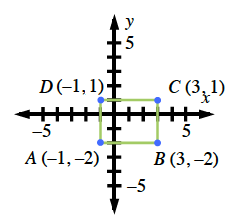### Home > GC > Chapter 3 > Lesson 3.1.1 > Problem3-5

3-5.

Plot the rectangle $ABCD$ formed with the points $A(−1, −2)$, $B(3, −2)$, $C(3, 1)$, and $D(−1, 1)$ onto graph paper. Use the method used in problem 3-2 to enlarge it from the origin by a factor of $2$ (using two “rubber bands”). Label this new rectangle $A'B'C'D'$.

1. What are the dimensions of the enlarged rectangle, $A'B'C'D'$?

The enlarged rectangle will be $6$ units by $8$ units.1. Find the area and the perimeter of $A'B'C'D'$.

The dimensions of the new rectangle will be factors of the original rectangle.

2. Find $AC$ (the length of $\overline {A C}$).

Think about how you would find the hypotenuse of a triangle.